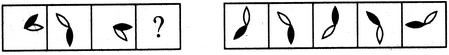# Non Verbal Reasoning - Analogy - Discussion

### Discussion :: Analogy - Section 1 (Q.No.21)

Each of the following questions consists of two sets of figures. Figures A, B, C and D constitute the Problem Set while figures 1, 2, 3, 4 and 5 constitute the Answer Set. There is a definite relationship between figures A and B. Establish a similar relationship between figures C and D by selecting a suitable figure from the Answer Set that would replace the question mark (?) in fig. (D).

21.

Select a suitable figure from the Answer Figures that would replace the question mark (?).

Problem Figures:                            Answer Figures:(A)     (B)      (C)     (D)                  (1)      (2)      (3)      (4)      (5)

 [A]. 1 [B]. 2 [C]. 3 [D]. 4 [E]. 5

Explanation:

The black leaf rotates 135oCW and the white leaf rotates 135oACW.

 Michael said: (Jun 25, 2013) How will you know how many degrees it will rotate?

 Usha said: (Dec 24, 2014) Tell me about angles, how it is?

 Hellan said: (Oct 10, 2021) Just think that there is an imaginary perpendicular like this "+" at the center.